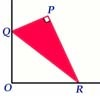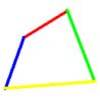You may also likeSet Square

A triangle PQR, right angled at P, slides on a horizontal floor with Q and R in contact with perpendicular walls. What is the locus of P?Biggest Bendy

Four rods are hinged at their ends to form a quadrilateral. How can you maximise its area?Strange Rectangle

ABCD is a rectangle and P, Q, R and S are moveable points on the edges dividing the edges in certain ratios. Strangely PQRS is always a cyclic quadrilateral and you can find the angles.

Consider any convex quadrilateral $Q$ made from four rigid rods with flexible joints at the vertices so that the shape of $Q$ can be changed while keeping the lengths of the sides constant. If the diagonals of the quadrilateral cross at an angle $\theta$ in the range $(0 \leq \theta < \pi/2)$, as we deform $Q$, the angle $\theta$ and the lengths of the diagonals will change.
Using the results of the two problems on quadrilaterals Diagonals for Area and Flexi Quads prove that the area of $Q$ is proportional to $\tan\theta$.